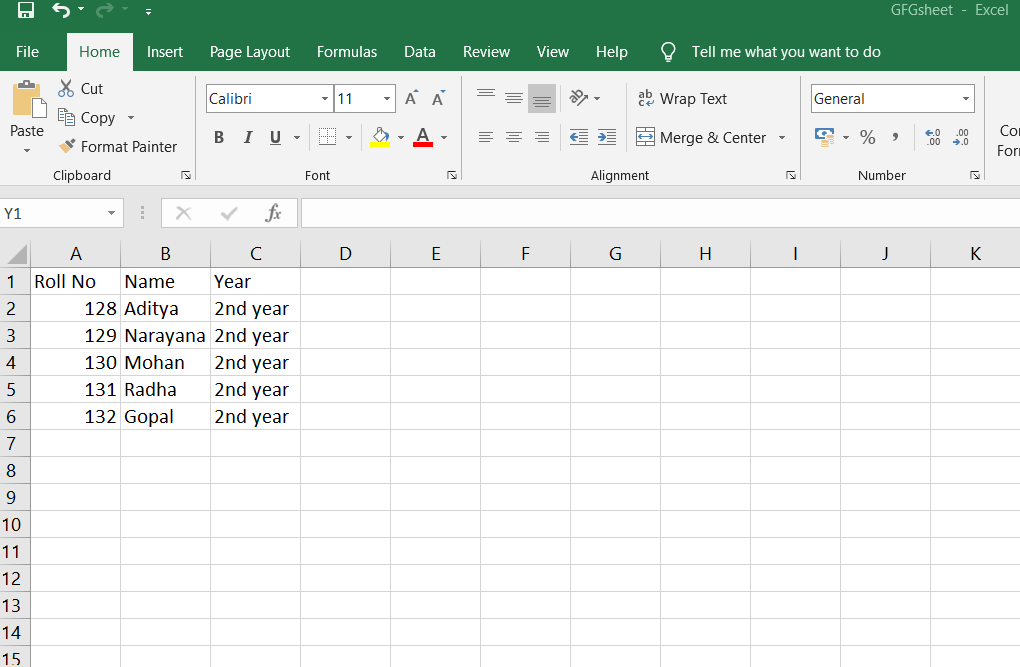Related Articles

# How to Write Data into Excel Sheet using Java?

• Difficulty Level : Medium
• Last Updated : 27 Nov, 2020

Handling files is an important part of any programming language. Java provides various in-built methods for creating, reading, updating, and deleting files. These methods are provided by the File class which is present in the java.io package. To perform file operations, Java uses the stream class.

To do operations in excel sheets using JAVA, it comes in handy to use the CSV files because CSV files can easily be used with Microsoft Excel, Google spreadsheets, and almost all other spreadsheets available.

To write data into an excel sheet itself using poi :

1. Create a blank workbook.

`XSSFWorkbook workbook = new XSSFWorkbook();`

2. Create a sheet and name it.

`XSSFSheet spreadsheet = workbook.createSheet(" Student Data ");`

3. Create a row

`Row row = sheet.createRow(rownum++);`

4. Add cells to the sheet.

5. Repeat Steps 3 and 4 to write the complete data.

Example:

## Java

 `// Java program to write data in excel sheet using java code`` ` `import` `java.io.File;``import` `org.apache.poi.ss.usermodel.Cell;``import` `org.apache.poi.xssf.usermodel.XSSFRow;``import` `org.apache.poi.xssf.usermodel.XSSFSheet;``import` `org.apache.poi.xssf.usermodel.XSSFWorkbook;``import` `java.io.FileOutputStream;``import` `java.util.Map;``import` `java.util.Set;``import` `java.util.TreeMap;`` ` `public` `class` `WriteDataToExcel {`` ` `    ``// any exceptions need to be caught``    ``public` `static` `void` `main(String[] args) ``throws` `Exception``    ``{``        ``// workbook object``        ``XSSFWorkbook workbook = ``new` `XSSFWorkbook();`` ` `        ``// spreadsheet object``        ``XSSFSheet spreadsheet``            ``= workbook.createSheet(``" Student Data "``);`` ` `        ``// creating a row object``        ``XSSFRow row;`` ` `        ``// This data needs to be written (Object[])``        ``Map studentData``            ``= ``new` `TreeMap();`` ` `        ``studentData.put(``            ``"1"``,``            ``new` `Object[] { ``"Roll No"``, ``"NAME"``, ``"Year"` `});`` ` `        ``studentData.put(``"2"``, ``new` `Object[] { ``"128"``, ``"Aditya"``,``                                            ``"2nd year"` `});`` ` `        ``studentData.put(``            ``"3"``,``            ``new` `Object[] { ``"129"``, ``"Narayana"``, ``"2nd year"` `});`` ` `        ``studentData.put(``"4"``, ``new` `Object[] { ``"130"``, ``"Mohan"``,``                                            ``"2nd year"` `});`` ` `        ``studentData.put(``"5"``, ``new` `Object[] { ``"131"``, ``"Radha"``,``                                            ``"2nd year"` `});`` ` `        ``studentData.put(``"6"``, ``new` `Object[] { ``"132"``, ``"Gopal"``,``                                            ``"2nd year"` `});`` ` `        ``Set keyid = studentData.keySet();`` ` `        ``int` `rowid = ``0``;`` ` `        ``// writing the data into the sheets...`` ` `        ``for` `(String key : keyid) {`` ` `            ``row = spreadsheet.createRow(rowid++);``            ``Object[] objectArr = studentData.get(key);``            ``int` `cellid = ``0``;`` ` `            ``for` `(Object obj : objectArr) {``                ``Cell cell = row.createCell(cellid++);``                ``cell.setCellValue((String)obj);``            ``}``        ``}`` ` `        ``// .xlsx is the format for Excel Sheets...``        ``// writing the workbook into the file...``        ``FileOutputStream out = ``new` `FileOutputStream(``            ``new` `File(``"C:/savedexcel/GFGsheet.xlsx"``));`` ` `        ``workbook.write(out);``        ``out.close();``    ``}``}`

Output:Attention reader! Don’t stop learning now. Get hold of all the important Java Foundation and Collections concepts with the Fundamentals of Java and Java Collections Course at a student-friendly price and become industry ready. To complete your preparation from learning a language to DS Algo and many more,  please refer Complete Interview Preparation Course.

My Personal Notes arrow_drop_up